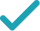Solve for a (complex solution)Solve for b (complex solution)Solve for aSolve for bSolve for xSteps Using the Quadratic Formula
Steps for Completing the Square
Solve for x (complex solution)Solve for cGraphStill have questions?
ax^{2}+c=-bx
Subtract bx from both sides. Anything subtracted from zero gives its negation.
ax^{2}=-bx-c
Subtract c from both sides.
x^{2}a=-bx-c
The equation is in standard form.
\frac{x^{2}a}{x^{2}}=\frac{-bx-c}{x^{2}}
Divide both sides by x^{2}.
a=\frac{-bx-c}{x^{2}}
Dividing by x^{2} undoes the multiplication by x^{2}.
a=-\frac{bx+c}{x^{2}}
Divide -bx-c by x^{2}.
bx+c=-ax^{2}
Subtract ax^{2} from both sides. Anything subtracted from zero gives its negation.
bx=-ax^{2}-c
Subtract c from both sides.
xb=-ax^{2}-c
The equation is in standard form.
\frac{xb}{x}=\frac{-ax^{2}-c}{x}
Divide both sides by x.
b=\frac{-ax^{2}-c}{x}
Dividing by x undoes the multiplication by x.
b=-ax-\frac{c}{x}
Divide -ax^{2}-c by x.
ax^{2}+c=-bx
Subtract bx from both sides. Anything subtracted from zero gives its negation.
ax^{2}=-bx-c
Subtract c from both sides.
x^{2}a=-bx-c
The equation is in standard form.
\frac{x^{2}a}{x^{2}}=\frac{-bx-c}{x^{2}}
Divide both sides by x^{2}.
a=\frac{-bx-c}{x^{2}}
Dividing by x^{2} undoes the multiplication by x^{2}.
a=-\frac{bx+c}{x^{2}}
Divide -bx-c by x^{2}.
bx+c=-ax^{2}
Subtract ax^{2} from both sides. Anything subtracted from zero gives its negation.
bx=-ax^{2}-c
Subtract c from both sides.
xb=-ax^{2}-c
The equation is in standard form.
\frac{xb}{x}=\frac{-ax^{2}-c}{x}
Divide both sides by x.
b=\frac{-ax^{2}-c}{x}
Dividing by x undoes the multiplication by x.
b=-ax-\frac{c}{x}
Divide -ax^{2}-c by x.
ax^{2}+bx+c=0
All equations of the form ax^{2}+bx+c=0 can be solved using the quadratic formula: \frac{-b±\sqrt{b^{2}-4ac}}{2a}. The quadratic formula gives two solutions, one when ± is addition and one when it is subtraction.
x=\frac{-b±\sqrt{b^{2}-4ac}}{2a}
This equation is in standard form: ax^{2}+bx+c=0. Substitute a for a, b for b, and c for c in the quadratic formula, \frac{-b±\sqrt{b^{2}-4ac}}{2a}.
x=\frac{\sqrt{b^{2}-4ac}-b}{2a}
Now solve the equation x=\frac{-b±\sqrt{b^{2}-4ac}}{2a} when ± is plus. Add -b to \sqrt{b^{2}-4ac}.
x=\frac{-\sqrt{b^{2}-4ac}-b}{2a}
Now solve the equation x=\frac{-b±\sqrt{b^{2}-4ac}}{2a} when ± is minus. Subtract \sqrt{b^{2}-4ac} from -b.
x=-\frac{\sqrt{b^{2}-4ac}+b}{2a}
Divide -b-\sqrt{b^{2}-4ac} by 2a.
x=\frac{\sqrt{b^{2}-4ac}-b}{2a} x=-\frac{\sqrt{b^{2}-4ac}+b}{2a}
The equation is now solved.
ax^{2}+bx+c=0
Quadratic equations such as this one can be solved by completing the square. In order to complete the square, the equation must first be in the form x^{2}+bx=c.
ax^{2}+bx+c-c=-c
Subtract c from both sides of the equation.
ax^{2}+bx=-c
Subtracting c from itself leaves 0.
\frac{ax^{2}+bx}{a}=-\frac{c}{a}
Divide both sides by a.
x^{2}+\frac{b}{a}x=-\frac{c}{a}
Dividing by a undoes the multiplication by a.
x^{2}+\frac{b}{a}x+\left(\frac{b}{2a}\right)^{2}=-\frac{c}{a}+\left(\frac{b}{2a}\right)^{2}
Divide \frac{b}{a}, the coefficient of the x term, by 2 to get \frac{b}{2a}. Then add the square of \frac{b}{2a} to both sides of the equation. This step makes the left hand side of the equation a perfect square.
x^{2}+\frac{b}{a}x+\frac{b^{2}}{4a^{2}}=-\frac{c}{a}+\frac{b^{2}}{4a^{2}}
Square \frac{b}{2a}.
x^{2}+\frac{b}{a}x+\frac{b^{2}}{4a^{2}}=\frac{b^{2}-4ac}{4a^{2}}